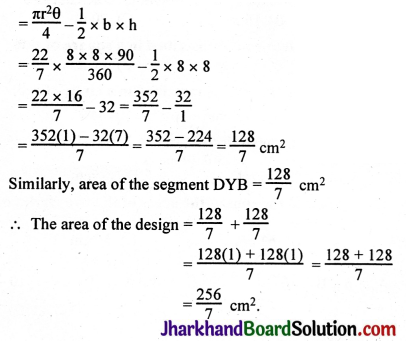## JAC Class 10 Maths Solutions Chapter 12 Areas Related to Circles Ex 12.2

Jharkhand Board JAC Class 10 Maths Solutions Chapter 12 Areas Related to Circles Ex 12.2 Textbook Exercise Questions and Answers.

## JAC Board Class 10 Maths Solutions Chapter 12 Areas Related to Circles Ex 12.2

Unless stated otherwise, use π = $$\frac{22}{7}$$

Question 1.
Find the area of a sector of a circle with radius 6 cm if angle of the sector is 60°.
Solution :
Area of a sector = $$\frac{\pi r^2 \theta}{360}$$
r = 6, θ = 60°
= $$\frac{22}{7} \times \frac{6 \times 6 \times 60}{360}=\frac{132}{7}$$ = 18.85 cm².Question 2.
Find the area of a quadrant of a circle whose circumference is 22 cm.
Solution :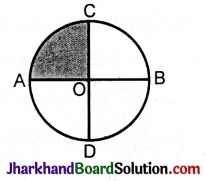С = 2πr = 22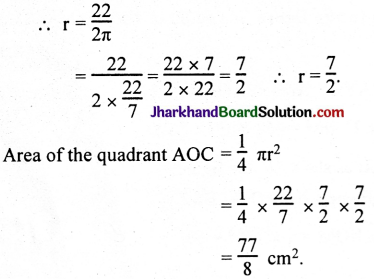Question 3.
The length of the minute hand of a clock is 14 cm. Find the area swept by the minute hand in 5 minutes.
Solution :
Length of the minute hand = 14 cm = r.
In 15 minutes the minute hand sweeps an area equal to a quadrant.
Area of the quadrant = $$\frac{\pi r^2}{4}$$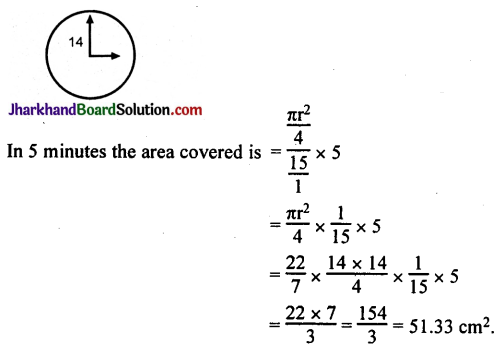Alternative Method:
One minute = 6°
5 minutes = 30°
Area swept by the minute hand
= πr² $$\frac{θ}{360°}$$
= $$\frac{22}{7}$$ × 14 × 14 × $$\frac{30°}{360°}$$
= 51.33 cm².

Question 4.
A chord of a circle of radius 10 cm subtends a right angle at the centre. Find the area of the corresponding: (i) minor segment, (ii) major sector. (Use π = 3.14)
Solution :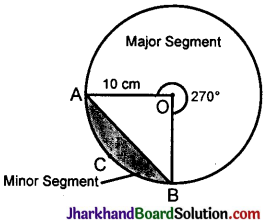Radius of the circle = 10 cm
Major segment is making 360° – 90° = 270°
Area of the sector making angle 270° = $$\frac{270°}{360°}$$ × πr² cm²
= $$\frac{1}{4}$$ × 10²π = 25 π cm²
= 25 × 3.14 cm² = 235.5 cm²
∴ Area of the major segment = 235.5 cm²
Height of ΔAOB = OA = 10 cm
Base of ΔAOB = OB = 10 cm
Area of ΔAOB = $$\frac{1}{2}$$ × OA × OB
= $$\frac{1}{2}$$ × 10 × 10 = 50 cm²
Major segment is making 90°
Area of the sector making angle 90° = $$\frac{90°}{360°}$$ × πr² cm²
= $$\frac{1}{4}$$ × 10²
= 25 × 3.14 cm² = 78.5 cm²
Area of the minor segment = Area of the sector making angle 90° – Area of ΔAOB
= 78.5 cm² – 50 cm² = 28.5 cm².Question 5.
In a circle of radius 21 cm, an arc subtends an angle of 60° at the centre. Find: (i) the length of the arc, (ii) area of the sector formed by the arc, (iii) area of the segment formed by the corresponding chord.
Solution :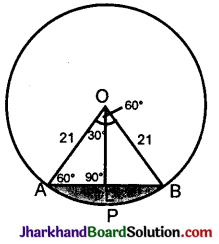(i) Length of the arc AB = $$\frac{2 \pi r \theta}{360^{\circ}}$$ θ = 60°, r = 21
= 2 × $$\frac{22}{7} \times \frac{21 \times 60}{360}$$ = 22cm

(ii) Area of the sector formed by the arc = $$\frac{\pi r^2 \theta}{360^{\circ}}$$
= $$\frac{22}{7} \times \frac{21 \times 21 \times 60}{360^{\circ}}$$
= 11 × 21 = 231 cm².

(iii) Area of the segment formed APBLA = Area of the sector PAOB – Area of the ΔOAB
Area of ΔOAB = ?
From O, draw OL ⊥ AB. AL = ?, OL = ?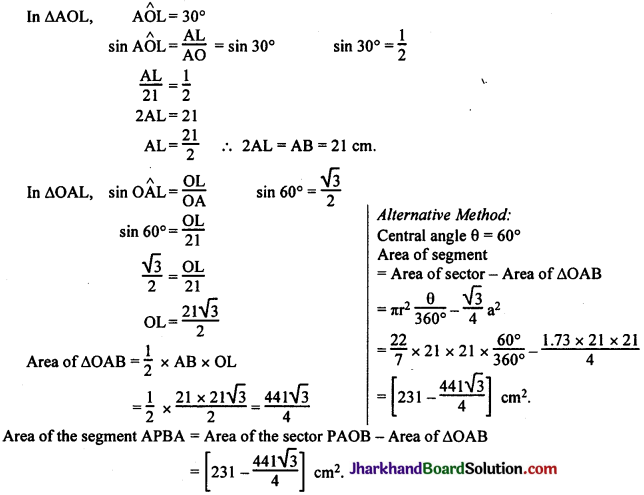Question 6.
A chord of a circle of radius 15 cm subtends an angle of 60° at the centre. Find the areas of the corresponding minor and major segments of the circle. (Use π = 3.14 and $$\sqrt{3}$$ = 1.73).
Solution :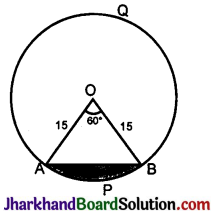Area of the minor segment APB = Area of the sector OAPB – Area of ΔOAB
= $$\frac{\pi r^2 \theta}{360^{\circ}}$$ – Area of ΔOAB
Area of the sector OAPB = $$\frac{3.14 \times 15 \times 15 \times 60}{360}$$ = 1.57 × 75 cm²
OPB is an isosceles triangle. OA = OB ∴ $$\hat{A}$$ = $$\hat{B}$$ = 60°.
The triangle becomes an equilateral triangle.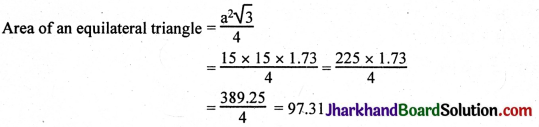Area of the sector OAPB = 1.57 × 75 = 117.75 cm²
∴ Area of the minor segment APB = 117.75 – 97.31 = 20.44 sq.cm.
Area of the major segment AQBA = Area of the circle – Area of the minor segment
= πr² – 20.44
= (3.14 × 15 × 15) – 20.44
= 706.50 – 20.44 = 686.06 sq.cm.Question 7.
A chord of a circle of radius 12 cm subtends an angle of 120° at the centre. Find the area of the corresponding segment of the circle. (Use π = 3.14 and $$\sqrt{3}$$ = 1.73).
Solution :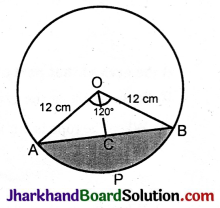Area of the segment APB = Area of the sector PAOB – Area of ΔOAB
r = 12, θ = 120°, π = 3.14
∴ Area of the sector PAOB = $$\frac{\pi r^2 \theta}{360^{\circ}}$$
= $$\frac{3.14 \times 12 \times 12 \times 120}{360}$$
= 3.14 × 12 × 4
= 3.14 × 48
= 150.72 cm².
Area of ΔOAB = $$\frac{1}{2}$$ × b × h b = AB, h = OC
Draw OC ⊥ AB.
AOB is an isosceles triangle. OC ⊥ AB.
ΔAOC ≅ BOC (RHS)
∴ AC = CB.
A$$\hat{O}$$C = 60°, O$$\hat{A}$$C = 30°, A$$\hat{C}$$O = 90°
In ΔOAC, O$$\hat{C}$$A = 90°. sin O$$\hat{A}$$C = $$\frac{OC}{OA}$$
sin 30° = $$\frac{OC}{12}$$ sin 30° = $$\frac{1}{2}$$
$$\frac{1}{2}$$ = $$\frac{OC}{12}$$
2OC = 12
OC = $$\frac{12}{2}$$ = 6 cm.

In ΔOAC, O$$\hat{C}$$A = 90°
OC² + AC² = OA²
6² + AC² = 12²
AC² = 12² – 6²
= (12 + 6) (12 – 6)
= 18 × 6 = 108
AC = $$\sqrt{108}$$ = $$\sqrt{36 \times 3}$$ = 6$$\sqrt{3}$$
AC = CB = 6$$\sqrt{3}$$
∴ AB = 12$$\sqrt{3}$$
Area of ΔOAB = $$\frac{1}{2}$$ × b × h
= $$\frac{1}{2}$$ × AB × OC
= $$\frac{1}{2}$$ × 12$$\sqrt{3}$$ × 6 = 36$$\sqrt{3}$$
Area of the segment APB = Area of the sector PAOB – Area of triangle OAB
= 150.72 – 62.28
= 88.44 cm².

Question 8.
A horse is tied to a peg at one corner of a square-shaped grass field of side 15 m by means of a 5 m long rope. Find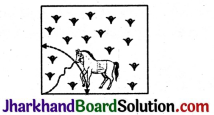(i) the area of that part of the field in which the horse can graze.
(ii) the increase in the grazing area if the rope were 10 m long instead of 5 m. (Use π = 3.14).
Solution :
(i) When the rope is 5m long, area grazed = $$\frac{\pi r^2}{4}$$ (quadrant)
= $$\frac{3.14 \times 5 \times 5}{4}=\frac{78.5}{4}$$ = 19.625 m².

(ii) When the rope is 10 m long, area grazed = $$\frac{\pi r^2}{4}$$
= $$\frac{3.14 \times 10 \times 10}{4}=\frac{3.14 \times 100}{4}=\frac{314}{4}$$
= 78.5 cm².
Increased area available when the rope is 10 m long is
= 78.500 – 19.625 = 58.875 cm².

Question 9.
A brooch is made with silver wire in the form of a circle with diameter 35 mm. The wire is also used in making 5 diameters which divide the circle into 10 equal sectors as shown in the figure. Find:
(i) the total length of the silver wire required
(ii) the area of each sector of the brooch.
Solution :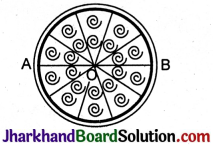A$$\hat{O}$$B = 180°
It is divided into five equal parts,
∴ Each part = θ = $$\frac{180°}{5}$$ = 36°.
Total length of silver wire used = πd × 5 × 35
= $$\frac{22}{7}$$ × 35 + 175
= 110 + 175
= 285 mm².

Area of each sector = $$\frac{\pi r^2 \theta}{360^{\circ}}$$
= $$\frac{22}{7} \times \frac{35}{2} \times \frac{35}{2} \times \frac{36}{360}$$
= $$\frac{11 \times 35}{4}=\frac{385}{4}$$ mm².Question 10.
An umbrella has 8 ribs which are equally spaced. Assuming umbrella to be a flat circle of radius 45 cm, find the area between two consecutive ribs of the umbrella.
Solution :
Number of ribs in umbrella = 8
Radius of umbrella while flat = 45 cm.
Angle between two consecutive ribs of the umbrella = $$\frac{360}{8}$$ = 45°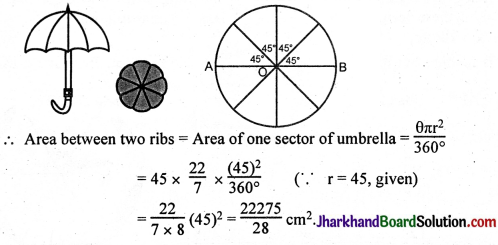Question 11.
A car has two wipers which do not overlap. Each wiper has a blade of length 25 cm sweeping through an angle of 115°. Find the total area cleaned at each sweep of the blades.
Solution :
Given, length of wiper blade = 25 cm = r (say)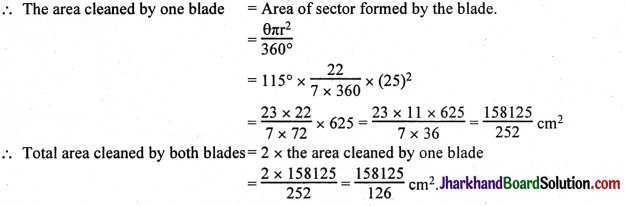Question 12.
To warn ships for underwater rocks, a lighthouse spreads a red coloured light over a sector of angle 80° to a distance of 16.5 km. Find the area of the sea over which the ships are warned. (Use π = 3.14)
Solution :
π = 3.14, r = 16.5, θ = 80°
Area warned = $$\frac{\theta \pi r^2}{360^{\circ}}$$
= $$\frac{3.14 \times 16.5 \times 16.5 \times 80}{360}$$
= 3.14 × 5.5 × 11
= 3.14 × 60.5 = 189.970
= 189.97 sq.kms.Question 13.
A round table cover has six equal designs as shown in the figure. If the radius of the cover is 28 cm, find the cost of making the designs at the rate of Re. 0.35 per cm². (Use $$\sqrt{3}$$ = 1.7).Solution :
[Draw a circle with centre O using a convenient radius. Draw a diameter AOD. Keep the protractor on AD and make three equal angles of 60° each such that A$$\hat{O}$$B = B$$\hat{O}$$C = C$$\hat{O}$$D. Produce BO and CO to meet the circumference at E and F respectively. Join AB, BC, CD, DE, EF, EA. We get a regular hexagon and six segments which are shaded.

Let one segment be APB.
Find the area of one segment. Multiply it by 6. We get the area of the six segments formed. Find the cost of making these designs as follows:
Area of one design × 6 × Rate]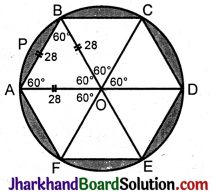The design APB is nothing but a segment. We have to find the area of this segment using the following relation:
Area of the sector OAPB – Area of equilateral ΔAOB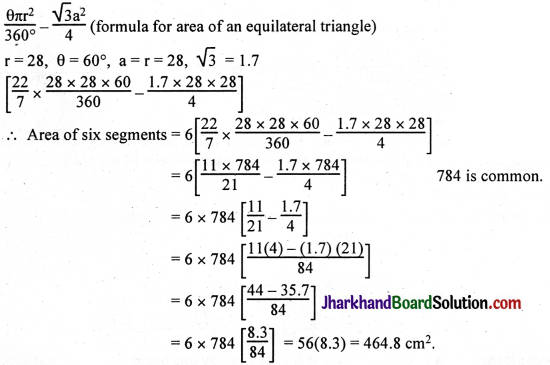Cost of making these six designs = Area × Rate
= 464.8 × 0.35
= Rs. 162.68.

Question 14.
Tick the correct answer in the following:
Area of a sector of angle p (in degrees) of a circle with radius R is
(A) $$\frac{P}{180}$$ × 2πR²
(B) $$\frac{P}{180}$$ × πR²
(C) $$\frac{P}{360}$$ × 2πR
(D) $$\frac{P}{720}$$ × 2πR²
Solution :
Area of sector = $$\frac{\theta \pi r^2}{360^{\circ}}$$ (Given, θ = p, r = R)
= $$\frac{p \pi R^2}{360}=\frac{p 2 \pi R^2}{720}$$

## JAC Class 10 Maths Solutions Chapter 12 Areas Related to Circles Ex 12.3

Jharkhand Board JAC Class 10 Maths Solutions Chapter 12 Areas Related to Circles Ex 12.3 Textbook Exercise Questions and Answers.

## JAC Board Class 10 Maths Solutions Chapter 12 Areas Related to Circles Exercise 12.3

Unless stated otherwise, use π = $$\frac{22}{7}$$

Question 1.
Find the area of the shaded region in the figure, if PQ = 24 cm, PR = 7 cm and O is the centre of the circle.Solution:
Given, PQ = 24 cm, PR = 7 cm.
We know any triangle drawn from diameter RQ to the circle is 90°.
Here, ∠RPQ = 90°
In right ΔRPQ, RQ2 = PR2 + PQ2 (By Pythagoras theorem)
RQ2 = 72 + 242
RQ2 = 49 + 576
RQ2 = 625
RQ = 25 cm
∴ Area of ΔRPQ = $$\frac{1}{2}$$ × RP × PQ
= $$\frac{1}{2}$$ × 7 × 24 = 84 cm2
∴ Area of semi-circle = $$\frac{\pi r^2}{2}=\frac{22}{7 \times 2}\left(\frac{25}{2}\right)^2$$ (∵ r = $$\frac{\mathrm{PQ}}{2}=\frac{25}{2}$$ cm)
= $$\frac{11 \times 625}{28}=\frac{6875}{28} \mathrm{~cm}^2$$
∴ Area of the shaded region = Area of the semi-circle – Area of right ΔRPQ
= $$\frac{6875}{28}-84$$
= $$\frac{6875-2352}{28}=\frac{4523}{28}$$ cm2.Question 2.
Find the area of the shaded region in the figure, if radii of the two concentric circles with centre O are 7 cm and 14 cm respectively and ∠AOC = 40°.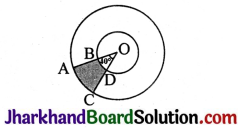Solution:
R – radius of the bigger circle, r – radius of the smaller circle.
Area of the shaded portion = Area of sector OAC – Area of sector OBD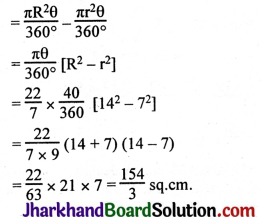Question 3.
Find the area of the shaded region in the figure, if ABCD is a square of side 14 cm and APD and BPC are semicircles.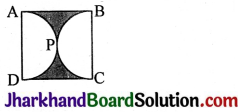Solution:
Area of the shaded portion = Area of the square – 2 × Area of one semicircle
= (14 × 14) – 2 × $$\frac{\pi r^2}{2}$$ [∵ r = 7]
= 14 × 14 – 2 × $$\frac{22}{7} \times \frac{7 \times 7}{2}$$
= 196 – 154 = 42 cm2.

Question 4.
Find the area of the shaded region in the figure, where a circular are of radius 6 cm has been drawn with vertex O of an equilateral triangle OAB of side 12 cm as centre.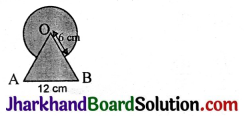Solution:
Area of the shaded portion = Area of the circle of radius 6 cm + Area of equilateral ΔABC – Area of the sector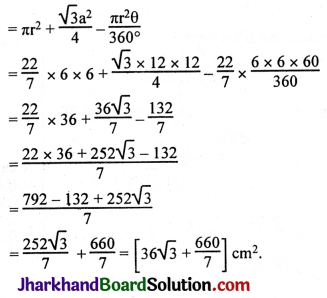Question 5.
From each corner of a square of side 4 cm a quadrant of a circle of radius 1 cm is cut and also a circle of diameter 2 cm is cut as shown in the figure. Find the area of the remaining portion of the square.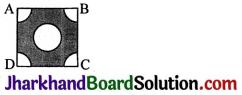Solution:
Area of the shaded portion = Area of the square – Area of the 4 quadrants – Area of the circle
= (4 × 4) – 4 × area of one quadrant – area of the circle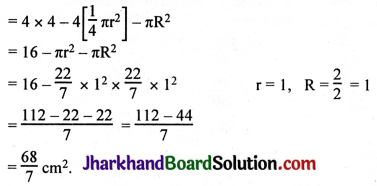Question 6.
In a circular table cover of radius 32 cm, a design is formed leaving an equilateral triangle ABC in the middle as shown in the figure. Find the area of the design.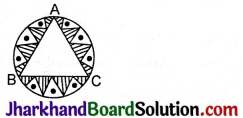Solution:
Area of the design (shaded region) = Area of the circle – Area of ΔABC
Area of equilateral triangle ABC = $$\frac{\sqrt{3} a^2}{4}$$
In ΔABC, AL ⊥ BC.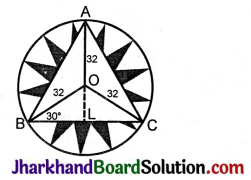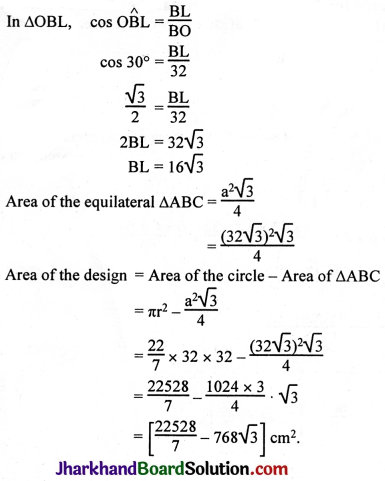Alternative Method: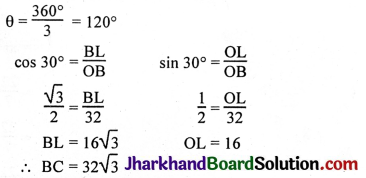= 3[Area of segments]
= 3[Area of sector – Area of ΔOBC]
= 3[$$\pi r^2 \frac{\theta}{360^{\circ}}-\frac{1}{2}$$ × BC × OL]

Question 7.
In the figure, ABCD is a square of side 14 cm. With centres A, B, C and D, four circles are drawn such that each circle touches externally two of the remaining three circles. Find the area of the shaded region.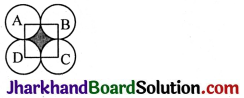Solution:
Area of the shaded region = Area of the square – Area of the 4 quadrants
= Area of the square – 4 × area of one quadrant
= (14)2 – 4 × $$\frac{1}{4}$$πr2
= (14)2 – 4 × $$\frac{1}{4} \times \frac{22}{7}$$ × 7 × 7
= 196 – 154
= 42 cm2.Question 8.
The figure depicts a racing track whose left and right ends are semicircular.
The distance between the two inner parallel line segments is 60 m and they are each 106 m. long. If the track is 10 m wide, find:
(i) the distance around the track along its inner edge.
(ii) the area of the track.Solution:
i) Distance around the inner track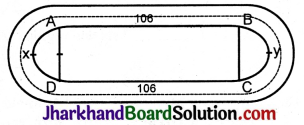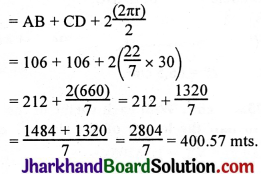ii) Area of the track: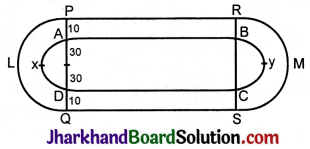Area of the track = l × b + l × b + 2$$\left[\frac{\pi \mathrm{R}^2}{2}-\frac{\pi \mathrm{r}^2}{2}\right]$$ r = 30 mts, R = (30 + 10) = 40 mts.
= 106 × 10 + 106 × 10 + 2 × $$\frac{\pi}{2}$$(R2 – r2)
= 1060 + 1060 + $$\frac{22}{7}$$[402 – 302]
= 2120 + $$\frac{22}{7}$$[1600 – 900]
= 2120 + $$\frac{22}{7}$$
= 2120 + 2200
= 4320 m2.

Question 9.
In the figure, AB and CD are two diameters of a circle (with centre O) perpendicular to each other and OD is the diameter of the smaller circle. If OA = 7 cm, find the area of the shaded region.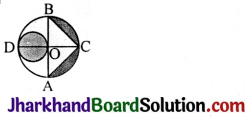Solution:
Given,
OA = 7 cm
∴ OD = 7 cm
Now, area of smaller circle whose diameter (OD) = 7 cm is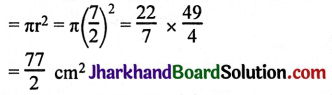Now,
Area of ΔABC = $$\frac{1}{2}$$ × AB × OC
= $$\frac{1}{2}$$ × 2 × OA × OC
= $$\frac{1}{2}$$ × 14 × 7 (∵ OA = OC)
= 49 cm2.
Area of semi-circle ABCA = $$\frac{\pi r^2}{2}=\frac{22}{7 \times 2}(7)^2$$
= 77 cm2
∴ Area of segments BC and AC = Area of semi-circle – Area of AABC
= 77 – 49 = 28 cm2
∴ Area of total shaded region = Area of small circle + Area of segments BC and AC
= $$\frac{77}{2}$$ + 28
= 38.5 + 28
= 66.5 cm2.Question 10.
The area of an equilateral triangle ABC is 17320.5 cm2. With each vertex of the triangle as centre, a circle is drawn with radius equal to half the length of the side of the triangle. Find the area of the shaded region. (Use π = 3.14 and $$\sqrt{3}$$ = 1.73205).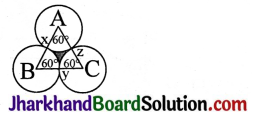Solution:
Area of the shaded portion = Area of equilateral ΔABC – Area of sector Axy – Area of sector Bxz – Area of sector Cyz.
π = 3.14, θ = 60°, r = ?, r = $$\frac{a}{2}$$
Area of the shaded portion = Area of the equilateral Δ – 3 × Area of one sector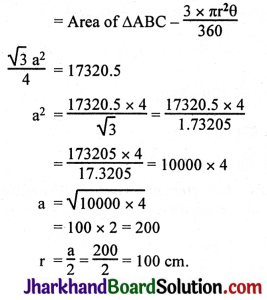Area of the shaded region = Area of ΔABC – $$\frac{3 \times \pi r^2 \theta}{360}$$
= 17320.5 – $$\frac{3 \times 3.14 \times 100 \times 100 \times 60}{360}$$
= 17320.5 – 1.57 × 10000
= 17320.5 – 15700.0
= 1620.5 sq.cm.

Question 11.
On a square handkerchief, nine circular designs each of radius 7 cm are made. Find the area of the remaining portion of the handkerchief.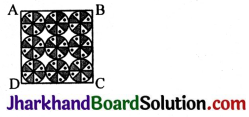Solution:
Number of circular designs = 9
Radius of the circular design = 7 cm
There are three circles in one side of the square handkerchief.
∴ Side of the square = 3 × diameter of circle = 3 × 14 = 42 cm
Area of the square = 42 × 42 cm2 = 1764 cm2
Area of the circle = πr2 = $$\frac{22}{7}$$ × 7 × 7 = 154 cm2
Total area of the design = 9 × 154 = 1386 cm2
Area of the remaining portion of the handkerchief = Area of the square – Total area of the design
= 1764 – 1386
= 378 cm2.

Question 12.
In the figure, OACB is a quadrant of a circle with centre O and radius 3.5 cm. If OD = 2 cm find the area of the (i) quadrant OACB, (ii) shaded region.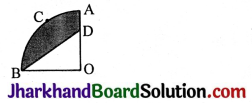Solution: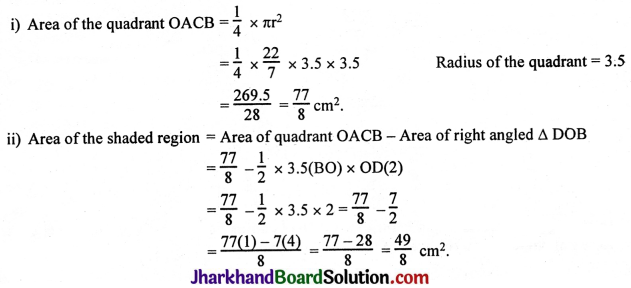Question 13.
In the figure, a square OABC is inscribed in a quadrant OPBQ. If OA = 20 cm, find the area of the shaded region. (Use π = 3.14)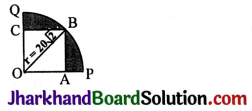Solution:
OB2 = OA2 + AB2
= 202 + 202
= 400 + 400
= 800
OB2 = 400 × 2
OB = $$\sqrt{400 \times 2}$$ = 20$$\sqrt{2}$$
Area of the shaded region = Area of the quadrant OPBQ – Area of the square OABC
= $$\frac{1}{4}$$ πr2 – (OA)2
= $$\frac{1}{4}$$ × 3.14 × 20$$\sqrt{2}$$ × 20$$\sqrt{2}$$ – (20)2
= $$\frac{1}{4}$$ × 3.14 × 400 × $$\sqrt{4}$$ – 400
= $$\frac{1}{4}$$ × 3.14 × 400 × 2 – 400
= 100 × 2 × 3.14 – 400
= 100 × 2(3.14 – 2)
= 200 × 1.14
= 228 sq.cm.Question 14.
AB and CD are respectively arcs of two concentric circles of radii 21 cm and 7 cm and centre O. If ∠AOB = 30°, find the area of the shaded region.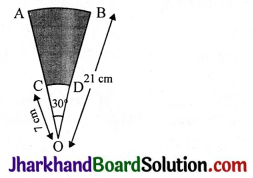Solution:
R = 21, r = 7, θ = 30°, π = $$\frac{22}{7}$$
Area of the shaded region = Area of sector OAB – Area of sector OCD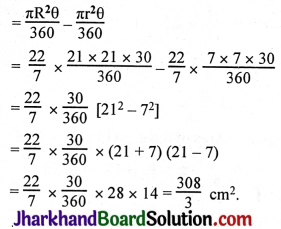Question 15.
In the figure, ABC is a quadrant of a circle of radius 14 cm and a semicircle is drawn with BC as diameter. Find the area of the shaded region.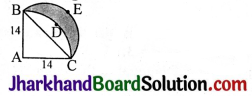Solution:
BC2 = 142 + 142
BC2 = 196 + 196 = 392
BC = $$\sqrt{392}$$ = $$\sqrt{196 \times 2}$$ = 14$$\sqrt{2}$$ = d
r = $$\frac{14 \sqrt{2}}{2}=7 \sqrt{2}$$
Radius of the sector = 14.
Area of the shaded region Area of the semicircle BEC – Area of the segment BDCB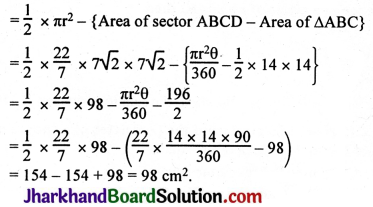Question 16.
Calculate the area of the designed region in the figure common between the two quadrants of circles of radius 8 cm each.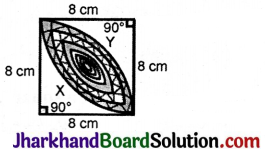Solution:
Area of the design = Area of sector DXB + Area of ΔDCB
Area of the segment DXB = Area of the sector DXBC – Area of ΔDCB